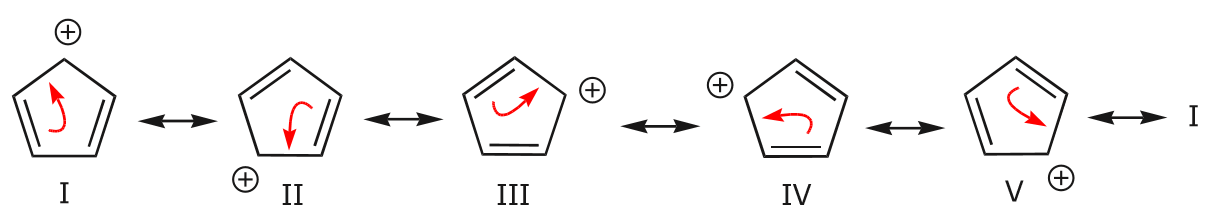# Is an antiaromatic compound more stable than a non-aromatic compound because of more conjugation?(image from a LibreTexts page (archive link))

It is shown that the antiaromatic compound is more stable than the non aromatic compounds 2 and 3 because of a more conjugated system. Here's the exact statement:

In the first structure, delocalization of the positive charge and the π bonds occurs over the entire ring. This becomes apparent when we look at all the possible resonance structures as shown below.In the second structure, delocalization is only possible over three carbon atoms. This is demonstrated by writing all the possible resonance forms below, which now number only two.Finally, the third structure has no delocalization of charge or electrons because no resonance forms are possible. Therefore, it is the least stable of the three.

Is this correct? I thought that the conjugation in an antiaromatic compound destabilized it more than a non aromatic compound.

• Good question! I highly doubt if this is true. But, I do not have the relevant data to post an answer. That said, I have also archived that page and added a link and the relevant paragraphs to your question, just in case the linked webpage changes and makes your question appear obsolete for future visitors. Mar 22, 2018 at 9:58
• It is often weird to compare the stability of species that differ by their formula anyway. The case is not as to compare a hypothetical localised cycloexatriene to benzene. ...but somehow to compare cyclohexane and benzene merely on demoralisation. Saying that Cyclopentadienyl cation is anti aromatic means it does not get planar. The non planar cation could be more stable than the other(s). But even in the case the explanation by the link is not correct. Delocalisation as depicted does not occur. Mar 22, 2018 at 11:00
• Demoralisation reads as delocalisation. T9 :)) And even non planar cyclopentadienyl cation is very unstable. Mar 22, 2018 at 11:08
• To be accurate, the stability of a compound depends on the exact configuration of the atoms. We have numerous theories telling a different story about the compound and all hold good for some and terribly wrong for some other. Chemistry is still a developing science and to look at it from the existing theories may lead to inaccurate results. May 14, 2019 at 15:10

Sometimes, only the delocalisation of electrons is not sufficient to conclude that the compound is more stable. We should also look at which molecular orbital the electron pair is delocalising. But a clear picture of these kind of $\pi$-conjugated systems can be got when it is approached quantum-mechanically, with the help of Huckel-$\pi$ Molecular Orbital Theory.
According to this theory, to get the $\pi$-electronic energy of the system, we have to solve the Secular Determinant, which is $$\ce{det(H_{ij}- ES_{ij}) =0 ,where i,j varies from 1 to n}$$ in a $n$ Carbon System.
$1$. $\ce{H_{ii} =$\alpha$for all i, and H_{ij} =$\beta$for |i -j|=1.}$ This approximation tells that the effective Hamiltonian for the whole conjugation is negligible if the carbons are not close to each other(i.e. adjacent).The two different values are obvious because of the in Hamiltonian, the Columb integral, the Exchange integral interferes.
$2$. $\ce{S_{ii} =1; and S_{ij}= 0 else}$. This tells the Overlap integral(Note that, this is an integral, and clear overlap in the picture doesn't mean integral should be large) are negligible for any two different carbons.
Taking these two approximations, if you solve the determinant, you will get energy levels for $5$ carbon systems,$$\ce{E = \alpha + 2\beta; \alpha; \alpha - \beta}$$Typically, the values of $\beta$ are negative and as you increase in $\alpha$ or decrease in $\beta$, the energy is increased and system gets destabilisation. .So, the lowest energy level is $\alpha$ + $2$$\beta, and then the second and after that the third one. Your first compound has four \pi electrons. So, two electrons will be in the level \alpha +2$$\beta$, and other two is in $\alpha$ only. So,net energy, is $4$$\alpha + 4$$\beta$ in mostly conjugated system $1$st compound.
But in the second case there are only two $\pi$ electrons. That two will go into $\alpha$ + $2$$\beta.So, net energy is 2$$\alpha$ + $4$$\beta$ in second one.
So, second system has lower energy than the first as coefficient of $\alpha$ is lesser in second one, though second compound is less conjugated. So, extent of delocalisation doesn't always determine stability.